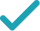Type a math problemFactorSolution Steps
Steps Using Direct Factoring Method
Solution Steps
Factor the expression by grouping. First, the expression needs to be rewritten as . To find and , set up a system to be solved.
Since is positive, and have the same sign. Since is negative, and are both negative. List all such integer pairs that give product .
Calculate the sum for each pair.
The solution is the pair that gives sum .
Rewrite as .
Factor out in the first and in the second group.
Factor out common term by using distributive property.
EvaluateGraph
a+b=-10 ab=3\times 8=24
Factor the expression by grouping. First, the expression needs to be rewritten as 3x^{2}+ax+bx+8. To find a and b, set up a system to be solved.
-1,-24 -2,-12 -3,-8 -4,-6
Since ab is positive, a and b have the same sign. Since a+b is negative, a and b are both negative. List all such integer pairs that give product 24.
-1-24=-25 -2-12=-14 -3-8=-11 -4-6=-10
Calculate the sum for each pair.
a=-6 b=-4
The solution is the pair that gives sum -10.
\left(3x^{2}-6x\right)+\left(-4x+8\right)
Rewrite 3x^{2}-10x+8 as \left(3x^{2}-6x\right)+\left(-4x+8\right).
3x\left(x-2\right)-4\left(x-2\right)
Factor out 3x in the first and -4 in the second group.
\left(x-2\right)\left(3x-4\right)
Factor out common term x-2 by using distributive property.
3x^{2}-10x+8=0
Quadratic polynomial can be factored using the transformation ax^{2}+bx+c=a\left(x-x_{1}\right)\left(x-x_{2}\right), where x_{1} and x_{2} are the solutions of the quadratic equation ax^{2}+bx+c=0.
x=\frac{-\left(-10\right)±\sqrt{\left(-10\right)^{2}-4\times 3\times 8}}{2\times 3}
All equations of the form ax^{2}+bx+c=0 can be solved using the quadratic formula: \frac{-b±\sqrt{b^{2}-4ac}}{2a}. The quadratic formula gives two solutions, one when ± is addition and one when it is subtraction.
x=\frac{-\left(-10\right)±\sqrt{100-4\times 3\times 8}}{2\times 3}
Square -10.
x=\frac{-\left(-10\right)±\sqrt{100-12\times 8}}{2\times 3}
Multiply -4 times 3.
x=\frac{-\left(-10\right)±\sqrt{100-96}}{2\times 3}
Multiply -12 times 8.
x=\frac{-\left(-10\right)±\sqrt{4}}{2\times 3}
x=\frac{-\left(-10\right)±2}{2\times 3}
Take the square root of 4.
x=\frac{10±2}{2\times 3}
The opposite of -10 is 10.
x=\frac{10±2}{6}
Multiply 2 times 3.
x=\frac{12}{6}
Now solve the equation x=\frac{10±2}{6} when ± is plus. Add 10 to 2.
x=2
Divide 12 by 6.
x=\frac{8}{6}
Now solve the equation x=\frac{10±2}{6} when ± is minus. Subtract 2 from 10.
x=\frac{4}{3}
Reduce the fraction \frac{8}{6}\approx 1.333333333 to lowest terms by extracting and canceling out 2.
3x^{2}-10x+8=3\left(x-2\right)\left(x-\frac{4}{3}\right)
Factor the original expression using ax^{2}+bx+c=a\left(x-x_{1}\right)\left(x-x_{2}\right). Substitute 2 for x_{1} and \frac{4}{3}\approx 1.333333333 for x_{2}.
3x^{2}-10x+8=3\left(x-2\right)\times \left(\frac{3x-4}{3}\right)
Subtract \frac{4}{3}\approx 1.333333333 from x by finding a common denominator and subtracting the numerators. Then reduce the fraction to lowest terms if possible.
3x^{2}-10x+8=\left(x-2\right)\left(3x-4\right)
Cancel out 3, the greatest common factor in 3 and 3.
x ^ 2 -\frac{10}{3}x +\frac{8}{3} = 0
Quadratic equations such as this one can be solved by a new direct factoring method that does not require guess work. To use the direct factoring method, the equation must be in the form x^2+Bx+C=0.This is achieved by dividing both sides of the equation by 3
r + s = \frac{10}{3} rs = \frac{8}{3}
Let r and s be the factors for the quadratic equation such that x^2+Bx+C=(x−r)(x−s) where sum of factors (r+s)=−B and the product of factors rs = C
r = \frac{5}{3} - u s = \frac{5}{3} + u
Two numbers r and s sum up to \frac{10}{3} exactly when the average of the two numbers is \frac{1}{2}*\frac{10}{3} = \frac{5}{3}. You can also see that the midpoint of r and s corresponds to the axis of symmetry of the parabola represented by the quadratic equation y=x^2+Bx+C. The values of r and s are equidistant from the center by an unknown quantity u. Express r and s with respect to variable u. <div style='padding: 8px'><img src='https://opalmath.azureedge.net/customsolver/quadraticgraph.png' style='width: 100%;max-width: 700px' /></div>
(\frac{5}{3} - u) (\frac{5}{3} + u) = \frac{8}{3}
To solve for unknown quantity u, substitute these in the product equation rs = \frac{8}{3}
\frac{25}{9} - u^2 = \frac{8}{3}
Simplify by expanding (a -b) (a + b) = a^2 – b^2
-u^2 = \frac{8}{3}-\frac{25}{9} = -\frac{1}{9}
Simplify the expression by subtracting \frac{25}{9} on both sides
u^2 = \frac{1}{9} u = \pm\sqrt{\frac{1}{9}} = \pm \frac{1}{3}
Simplify the expression by multiplying -1 on both sides and take the square root to obtain the value of unknown variable u
r =\frac{5}{3} - \frac{1}{3} = 1.333 s = \frac{5}{3} + \frac{1}{3} = 2.000
The factors r and s are the solutions to the quadratic equation. Substitute the value of u to compute the r and s.